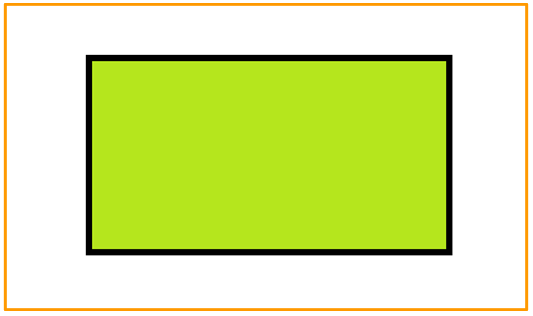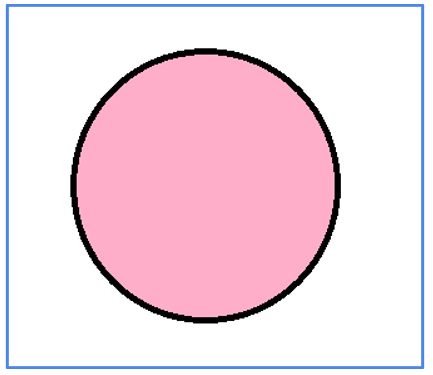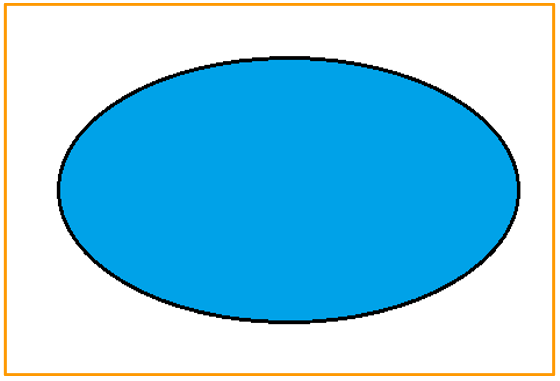# Image Map Area in HTML

## Image Map Area in HTML with Examples

In this article, I am going to discuss Image Map Area in HTML with Examples. Please read our previous article where we discussed Image Maps in HTML with Examples. At the end of this article, you will learn everything about HTML Image Map Area with Examples.

##### HTML Imagemap Area

The HTML <area> element specifies a predetermined clickable area within an image map. Geometric areas on an image can be directly linked with hypertext links using an image map.

Inside the area element coords attribute is used to define the coordinates of a clickable object inside the image and the shape attribute is used to define the shape of a clickable area for example rectangle, circle, etc.

##### Rectangle

Rectangle coords have the values x1,y1,x2,y2. The coordinates of the rectangle’s top-left and bottom-right corners are specified by this value.

coords=” 60,40,391,195 “

###### Example
```<!DOCTYPE html>
<html>
<body>

<map name="rectmap">
<area shape="rect" coords="60,40,391,195" alt="rectangle" href="https://en.wikipedia.org/wiki/Rectangle">
</map>

</body>
</html>
```

When you run the above HTML code, you will get the following output in the browser.The coordinates are 60, 40, and 391,195 specifying the top-left and bottom-right corners of the rectangle respectively.

##### Circle

Circle coords have the value x,y, radius.The coordinates of the circle center and the radius are specified by this value.

coords=” 151,147,120 “

###### Example
```<!DOCTYPE html>
<html>
<body>

<map name="circlemap">
<area shape="circle" coords="151,147,120" alt="circle" href="https://en.wikipedia.org/wiki/Circle">
</map>

</body>
</html>
```

When you run the above HTML code, you will get the following output in the browser.The coordinates are 151, 147, and 120 specifying the circle center and radius respectively.

##### Polygon

Polygon coords have the values x1,y1,x2,y2,..,xn,yn. The coordinates of the edges of the polygon are specified by this value. The browser will add the last coordinate pair to close the polygon if the first and last coordinate pairs are not the same.

coords=” 48,163,260,56,465,166,267,270,48,163 “

###### Example
```<!DOCTYPE html>
<html>
<body>

<map name="polymap">
<area shape="poly" coords="48,163,260,56,465,166,267,270,48,163" alt="polygon" href="https://en.wikipedia.org/wiki/Polygon">
</map>

</body>
</html>
```

When you run the above HTML code, you will get the following output in the browser.In the next article, I am going to discuss HTML Tables with Examples. Here, in this article, I try to explain Image Map Area in HTML with Examples and I hope you enjoy this HTML Image Maps with Examples article.